Courses

# Ionic Equation MCQ - 1 (Advance)

## 20 Questions MCQ Test Chemistry for JEE Advanced | Ionic Equation MCQ - 1 (Advance)

Description
This mock test of Ionic Equation MCQ - 1 (Advance) for JEE helps you for every JEE entrance exam. This contains 20 Multiple Choice Questions for JEE Ionic Equation MCQ - 1 (Advance) (mcq) to study with solutions a complete question bank. The solved questions answers in this Ionic Equation MCQ - 1 (Advance) quiz give you a good mix of easy questions and tough questions. JEE students definitely take this Ionic Equation MCQ - 1 (Advance) exercise for a better result in the exam. You can find other Ionic Equation MCQ - 1 (Advance) extra questions, long questions & short questions for JEE on EduRev as well by searching above.
*Multiple options can be correct
QUESTION: 1

### A 1 litre solution of pH = 1 diluted upto 10 times. What volume of a solution with pH = 2 is to be added in diluted solution so that pH does not change :

Solution:

After dilution [H+] = 10-2 ⇒ pH = 2

Let V litre solution of pH = 2 is added in original solution so that pH remains fixed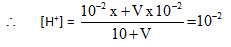This result is independent of volume taken.

*Multiple options can be correct
QUESTION: 2

### The solubility of a sparingly soluble salt A. By in water at 25°C 1.4 x 10-4 M. The solubility product is 1.1 x 10-11. The possibilities are :

Solution:

KSP = 1.1 x 10-11 = (1.4 * 10-4)x + y xx  - yyso we have x + y = 3 (by comparing values)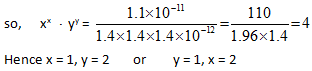*Multiple options can be correct
QUESTION: 3

### The variation of pH during the titration of 0.5 N Na2CO3 with 0.5 N HCI is shown in the given graph. The following table indicates the colour and pH ranges of different indicators :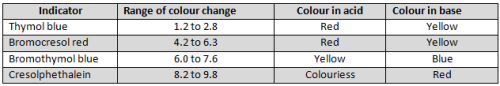Based on the graph and the table, which of the following statements are true?

Solution:
*Multiple options can be correct
QUESTION: 4

Which of the following solutions when added to 1L of a 0.1 M CH3COOH solution will cause no change in either the degree of dissociation of CH3COOH or the pH of the solution. Ka = 1.8 x 10-5 for CH3COOH?

Solution: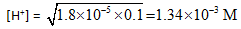to which the solution in (C & D) are ISOHYDRIC.

*Multiple options can be correct
QUESTION: 5

Buffer solution A of a weak monoprotic acid and its sodium salt in the concentration ratio x : y has pH = (pH),. Buffer solution B of the same acid and its sodium salt in the concentration ratio y : x has pH = (pH)2. If (pH)2— (pH), =1 unit and (pH), + (pH)2 = 9.5 units, then

Solution:

(pH)1 = pKa + log (y/x)

(pH)2 = pKa + log (x/y)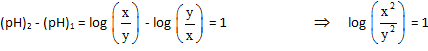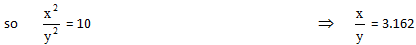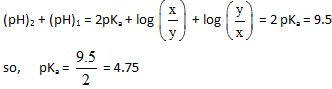QUESTION: 6

Radiochemical techniques can be used to determine solubility product eslimation. The measurement of radioactivity can be used to find the concentration in a solubthty equilibrium giving afak idea about  various equilibrium concentrations. In an experiment, 50.00m1 of a 0.010 81A*1103sdufion containing a silver isotope with a radioactivity of 75,000 counts per min per mi were mixed viii 100 ml of a 0.03 Na103 solution. The mixed solution was diluted 500 mi and filtered to remove al oftheAg/03predpilateleavinig behind a radioactive solution. Molar mass of AgI03 = 285 g/mol.

Q.

The remaining solution was found to have a radioactivity of 50 cane tsper min per mi. Then find the mass of the ppt of AgI03 obtained separated.

Solution:

Moles of AgIO3 precipitated = 149/150 millimoles

Mass of AgIO3 precipitated =285x149/150 x10-3 = 0.283 g

QUESTION: 7

Radiochemical techniques can be used to determine solubility product eslimation. The measurement of radioactivity can be used to find the concentration in a solubthty equilibrium giving afak idea about  various equilibrium concentrations. In an experiment, 50.00m1 of a 0.010 81A*1103sdufion containing a silver isotope with a radioactivity of 75,000 counts per min per mi were mixed viii 100 ml of a 0.03 Na103 solution. The mixed solution was diluted 500 mi and filtered to remove al oftheAg/03predpilateleavinig behind a radioactive solution. Molar mass of AgI03 = 285 g/mol.

Q.

The Ksp of AgI03 silver(radioactive) are :

Solution:

KSP = [Ag+][IO3-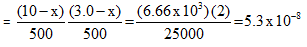QUESTION: 8

Radiochemical techniques can be used to determine solubility product eslimation. The measurement of radioactivity can be used to find the concentration in a solubthty equilibrium giving afak idea about  various equilibrium concentrations. In an experiment, 50.00m1 of a 0.010 81A*1103sdufion containing a silver isotope with a radioactivity of 75,000 counts per min per mi were mixed viii 100 ml of a 0.03 Na103 solution. The mixed solution was diluted 500 mi and filtered to remove al oftheAg/03predpilateleavinig behind a radioactive solution. Molar mass of AgI03 = 285 g/mol.

Q.

The % of unprecipitated silver ions is

Solution: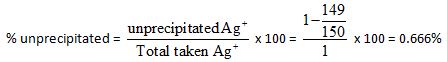QUESTION: 9

Consider a solution of CH3COONH4 which is a salt of weak acid &weak base. The

equilibrium involved in the solutions are :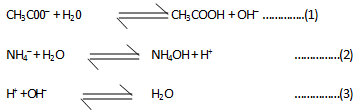If we add these three reactions, then the net reaction is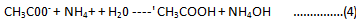Both CH3C00- and NH4' get hydrolysed independently and their hydrolysis depends on

(i) their initial concentration

(ii) the value of Kh which is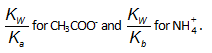Since both of the ions were produced from the same salt, their initial concentrations are same . Therefore unless
& untial the value of  Kw/Kand Kb is same, the degree of hydrolysis of ion can't be same.

To explain why we assume that degree of hydrolysis of cation and anion is same, we need to now look at the third reaction i.e., combination of H+ and OH – ions. It is obvious that this reaction happens only because one reaction produced H+ ion and the other produced OH– ions. We can also note that this reaction causes both the hydrolysis reaction to occur more since their product ions are being consumed. Keep this thing in mind that the equilibrium which has smaller value of equilibrium conxtant is affected more by the common ion effect. For the same reason if for any reason a reaction is made to occur to a greater extent by the consumption of any one of the product ion, the reaction with the smaller value of equilibrium constant tends to get affected more.

Therefore we conclude that firstly the hydrolysis of both the ions ocurs more in the presence of each other (due to consumption of the product ions) than in each other is absence. Secondly the hydrolysis of the ion which occurs to a lesser extent (due to smaller value of Kh) is affected more than the one whose Kh is greater. Hence we can see that the degree of hydrolysis of both the ions would be close to each other when they are getting hydrolysed in the presence of each other.

Q.

In the hydrolysis of salt of weak acid & weak base :

Solution:
QUESTION: 10

For 0.1 M CH3COONH4 salt solution given,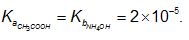In this case : degree of hydrolysis of cation and anion is

Solution:

Ka=Kb

QUESTION: 11

Consider a solution of CH3COONH4 which is a salt of weak acid &weak base. The

equilibrium involved in the solutions are :If we add these three reactions, then the net reaction isBoth CH3C00- and NH4' get hydrolysed independently and their hydrolysis depends on

(i) their initial concentration

(ii) the value of Kh which isSince both of the ions were produced from the same salt, their initial concentrations are same . Therefore unless
& untial the value of  Kw/Kand Kb is same, the degree of hydrolysis of ion can't be same.

To explain why we assume that degree of hydrolysis of cation and anion is same, we need to now look at the third reaction i.e., combination of H+ and OH – ions. It is obvious that this reaction happens only because one reaction produced H+ ion and the other produced OH– ions. We can also note that this reaction causes both the hydrolysis reaction to occur more since their product ions are being consumed. Keep this thing in mind that the equilibrium which has smaller value of equilibrium conxtant is affected more by the common ion effect. For the same reason if for any reason a reaction is made to occur to a greater extent by the consumption of any one of the product ion, the reaction with the smaller value of equilibrium constant tends to get affected more.

Therefore we conclude that firstly the hydrolysis of both the ions ocurs more in the presence of each other (due to consumption of the product ions) than in each other is absence. Secondly the hydrolysis of the ion which occurs to a lesser extent (due to smaller value of Kh) is affected more than the one whose Kh is greater. Hence we can see that the degree of hydrolysis of both the ions would be close to each other when they are getting hydrolysed in the presence of each other.

Q.

In a solution of NaHCO3 , the amphiprotic anion can under ionization to form H+ ion and hydrolysis to from OH ion.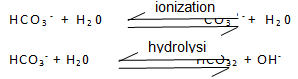To calculat PH, suitable approximation is :

Solution: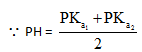QUESTION: 12

When aluminium salts are added to water, Ae3+ ions are immediately attracted to the negative end of polar water molecules. They form hexaaquaaluminum(III) ions, [Al(H20)6]3+. This is often written simply as Al+3(aq). However the electric field associated with small, highly charged Al+3 ion is so intense that it draws electrons in the 0 – H bonds of water towards itself. This enables the water molecules to become donors. In aqueous solution, free water molecules act as bases and the following equilibrium is established ;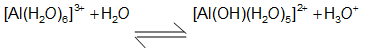Thus, solutions of Al+3 salts are acidic, in fact as acidic as vinegar. When a base stronger than H2O, e.g. S2-is added to aqueous aluminium salts further H+ ions are removed from [Al(H20)6]3+ and insoluble aluminium hydroxide precipitates.

2[Al(H20)6]3+ (aq) + 3S2- (aq)  → 2[Al(OH)3(H20)3](s) + 3H2S(g)

[Al(H20)6]3+ + 40H–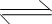[ Al(OH)4(H20)] (aq) + 4H20(l)

Q.

Which of [Al(H2O)6]3+, H2S or H20 is the strongest acid ?

Solution:
QUESTION: 13

When aluminium salts are added to water, Ae3+ ions are immediately attracted to the negative end of polar water molecules. They form hexaaquaaluminum(III) ions, [Al(H20)6]3+. This is often written simply as Al+3(aq). However the electric field associated with small, highly charged Al+3 ion is so intense that it draws electrons in the 0 – H bonds of water towards itself. This enables the water molecules to become donors. In aqueous solution, free water molecules act as bases and the following equilibrium is established ;Thus, solutions of Al+3 salts are acidic, in fact as acidic as vinegar. When a base stronger than H2O, e.g. S2-is added to aqueous aluminium salts further H+ ions are removed from [Al(H20)6]3+ and insoluble aluminium hydroxide precipitates.

2[Al(H20)6]3+ (aq) + 3S2- (aq)  → 2[Al(OH)3(H20)3](s) + 3H2S(g)

[Al(H20)6]3+ + 40H–[ Al(OH)4(H20)] (aq) + 4H20(l)

Q.

A base which will behave just like S2- :

Solution:
QUESTION: 14

When aluminium salts are added to water, Ae3+ ions are immediately attracted to the negative end of polar water molecules. They form hexaaquaaluminum(III) ions, [Al(H20)6]3+. This is often written simply as Al+3(aq). However the electric field associated with small, highly charged Al+3 ion is so intense that it draws electrons in the 0 – H bonds of water towards itself. This enables the water molecules to become donors. In aqueous solution, free water molecules act as bases and the following equilibrium is established ;Thus, solutions of Al+3 salts are acidic, in fact as acidic as vinegar. When a base stronger than H2O, e.g. S2-is added to aqueous aluminium salts further H+ ions are removed from [Al(H20)6]3+ and insoluble aluminium hydroxide precipitates.

2[Al(H20)6]3+ (aq) + 3S2- (aq)  → 2[Al(OH)3(H20)3](s) + 3H2S(g)

[Al(H20)6]3+ + 40H–[ Al(OH)4(H20)] (aq) + 4H20(l)

Q.

Another ion that would behave similar to AI+3 (aq) in forming an acidic salvation:

Solution:
QUESTION: 15

When aluminium salts are added to water, Ae3+ ions are immediately attracted to the negative end of polar water molecules. They form hexaaquaaluminum(III) ions, [Al(H20)6]3+. This is often written simply as Al+3(aq). However the electric field associated with small, highly charged Al+3 ion is so intense that it draws electrons in the 0 – H bonds of water towards itself. This enables the water molecules to become donors. In aqueous solution, free water molecules act as bases and the following equilibrium is established ;Thus, solutions of Al+3 salts are acidic, in fact as acidic as vinegar. When a base stronger than H2O, e.g. S2-is added to aqueous aluminium salts further H+ ions are removed from [Al(H20)6]3+ and insoluble aluminium hydroxide precipitates.

2[Al(H20)6]3+ (aq) + 3S2- (aq)  → 2[Al(OH)3(H20)3](s) + 3H2S(g)

[Al(H20)6]3+ + 40H–[ Al(OH)4(H20)] (aq) + 4H20(l)

Q.

[Al(OH)3 (H20)3] (s) more simply written as Al(OH)3(s) is :

Solution:
QUESTION: 16

When aluminium salts are added to water, Ae3+ ions are immediately attracted to the negative end of polar water molecules. They form hexaaquaaluminum(III) ions, [Al(H20)6]3+. This is often written simply as Al+3(aq). However the electric field associated with small, highly charged Al+3 ion is so intense that it draws electrons in the 0 – H bonds of water towards itself. This enables the water molecules to become donors. In aqueous solution, free water molecules act as bases and the following equilibrium is established ;Thus, solutions of Al+3 salts are acidic, in fact as acidic as vinegar. When a base stronger than H2O, e.g. S2-is added to aqueous aluminium salts further H+ ions are removed from [Al(H20)6]3+ and insoluble aluminium hydroxide precipitates.

2[Al(H20)6]3+ (aq) + 3S2- (aq)  → 2[Al(OH)3(H20)3](s) + 3H2S(g)

[Al(H20)6]3+ + 40H–[ Al(OH)4(H20)] (aq) + 4H20(l)

Q.

Which gas would get absorbed when passed into a solution of A!+3 (aq) ?

Solution:
QUESTION: 17

Match the following :

If ka of HCN = 5 x 10-10 , ka of HOCN = 3.2 x 10-4 , kb of NH3 = 1.8 x 10-5 , ka of CH3COOH = 1.8 x 105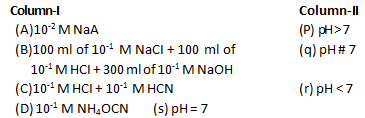Solution:
QUESTION: 18

Match the effect of addition of 0.1 M KOH to 0.1 M, 50 ml H3PO4 Ka1, Ka2 Ka3 are the I, II, III ionisation constant of H3PO4 :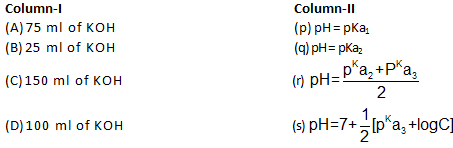Solution:
QUESTION: 19

If we mix equal volume of two solution. Match the following :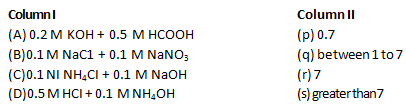Solution:

(A) acidic buffer will form,

(B ) both are salt of SASB,

(C) weak base NH4OH will form,

(D) remain strong acid solution

QUESTION: 20

K1 and K2 are first and second ionisation constant of H2CrO4, K3 is dissociation constant for NH3.

Match the following: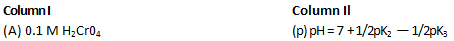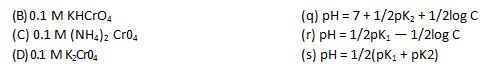Solution:

(A) diprotic weak acid, H+ only comes from first ionization (K1)

(B) HCrO4 is amphiprotic anion.

(C) (NH4)2 CrO4 it is salt of weak base and weak acid.

(D) Hydolysis of CrO42– (polyvalent anion), but only first hydrolysis is apprecitable.